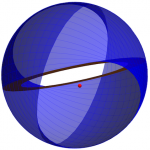The free electron is a two-dimensional current disc of zero thickness comprising a continuum of azimuthal circular currents elements confined to a limiting disc radius in the xy-plane that matches the de Broglie wavelength. These circular current elements transform into a semispherical basis element current vector field (BECVF) comprising great circle current elements, and the BECVF in turn serves as a basis element to form a uniform two-dimensional spherical shell of current of zero thickness. The current motion of the free electron gives rise to angular momentum vectors with projections of Lz = hbar, Lx = Ly = 0. The current motion of the bound electron gives rise to angular momentum vectors with projections of Lz = hbar/2, Lx = -Ly = hbar/4. The precession of either electron state in a magnetic field gives rise to a Bohr magneton of magnetic moment along the applied field axis that can only be parallel or antiparallel to the magnetic field wherein flux of the magnetic flux quantum (h/2e) is linked in the quantized spin transition in order to conserve angular momentum. The corresponding energy of the flux linkage gives rise to the electron g factor which is derived to an accuracy only limited by the accuracy of the fine structure constant of the exact solution of g.
The exact equations of the bound and free electron are given in Chapters 1 and 3 of Mills GUTCP, respectively, [https://brilliantlightpower.com/book-download-and-streaming/].

View the animation by clicking on the graphic or here.